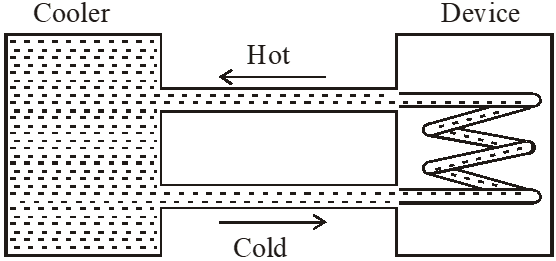Download the eSaral app and start learning from Kota's top IITians and doctors.

# Calorimetry - JEE Advanced Previous Year Questions with Solutions

JEE Advanced Previous Year Questions of Physics with Solutions are available at eSaral. Practicing JEE Advanced Previous Year Papers Questions of Physics will help the JEE aspirants in realizing the question pattern as well as help in analyzing weak & strong areas. Get detailed Class 11th & 12th Physics Notes to prepare for Boards as well as competitive exams like IIT JEE, NEET etc. eSaral helps the students in clearing and understanding each topic in a better way. eSaral is providing complete chapter-wise notes of Class 11th and 12th both for all subjects.     Click Here for JEE main Previous Year Topic Wise Questions of Physics with Solutions    Download eSaral app  for free study material and video tutorials.     Simulator   Previous Years JEE Advanced Questions
Q. A piece of ice (heat capacity = 2100 J $\mathrm{kg}^{-1}$ $^{\circ} \mathrm{C}^{-1}$ and latent heat = 3.36 × $10^{5} \mathrm{J} \mathrm{kg}^{-1}$) of mass m grams is at $-5^{\circ}$C at atmospheric pressure. It is given 420 J of heat so that the ice starts melting. Finally when the ice–water mixture is in equilibrium, it is found that 1 gm of ice has melted. Assuming there is no other heat exchange in the process, the value of m is ? [JEE 2010]
Ans. 8 $420=\left(m \times 10^{-3}\right)(2100)(5)+\left(1 \times 10^{-3}\right) \times\left(3.36 \times 10^{5}\right)$ $m=8 \mathrm{gm}$
Q. A water cooler of storage capacity 120 litres can cool water at constant rate of P watts. In a closed circulation system (as shown schematically in the figure), the water from the cooler is used to cool an external device that generates constantly 3 kW of heat (thermal load). The temperature of water fed into the device cannot exceed $30^{\circ}$C and the entire stored 120 litres of water is initially cooled to 10°C. The entire system is thermally insulated. The minimum value of P (in watts) for which the device can be operated for 3 hours is :(Specific heat of water is $4.2 \mathrm{kJ} \mathrm{kg}^{-1} \mathrm{K}^{-1}$ and the density of water is $1000 \mathrm{kg} \mathrm{m}^{-3}$ ) (A) 1600 (B) 2067 (C) 2533 (D) 3933 [JEE-Advance-2016]
Ans. (B) $3000-\mathrm{P}=(120 \times 1)\left(4.2 \times 10^{3}\right) \frac{\mathrm{dT}}{\mathrm{dt}}$ $\frac{\mathrm{dT}}{\mathrm{dt}}=\frac{20}{60 \times 60 \times 3}$ $\mathrm{P}=2067 \mathrm{W}$